# 线性滤波算法的机器视觉适用性研究Research on the Machine Vision Applicability of Linear Filtering Algorithm

DOI: 10.12677/CSA.2020.103041, PDF, HTML, XML, 下载: 259  浏览: 492  科研立项经费支持

Abstract: In the actual working process of machine vision system, due to the diversity of state noise, it is difficult to choose the best filtering algorithm in real time. In this paper, the linear filtering algorithm: Instant Domain Recursive filtering (IDR), Gaussian filtering, Laplace of Gaussian filtering (LOG), Binomial Coefficient filtering (BC) algorithm model, calculation speed and output results are studied in depth. The performance of the above algorithm is tested by superposition random noise combination, and its applicability in engineering practice is analyzed. The experimental results show that Gaussian filtering achieves the best compromise between time and accuracy when white noise and Gaussian noise are superimposed; when salt and pepper noise are superimposed, Gaussian Laplace filtering is obviously superior to the other three algorithms, providing theoretical and technical support for the optimization of image processing effect of machine vision system.

1. 引言

2. 使用须知

2.1. 时域递归滤波

${I}_{1}={I}_{1}+k\left({I}_{0}-{I}_{1}\right)$ (1)

$H\left(z\right)=\frac{1-k}{1-k{z}^{-1}}$ (2)

${|H\left(\omega \right)|}^{2}=\frac{{\left(1-k\right)}^{2}}{1+{k}^{2}-2k\mathrm{cos}\omega }$ (3)

$\begin{array}{cc}{I}_{1}={I}_{1}& k=0\\ {I}_{1}={I}_{0}& k=1\end{array}\right\}$ (4)

$P=10g\frac{1+k}{1-k}$ (5)

2.2. 高斯滤波(6)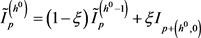(7)(8)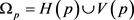(9)

2.3. 高斯–拉普拉斯滤波

LOG算法来源于Marr提出的边缘检测思想其对图像的平滑采用二维高斯函数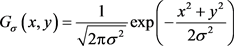(10)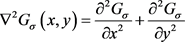(11)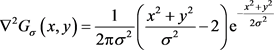(12)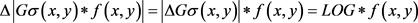(13)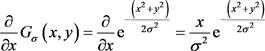(14)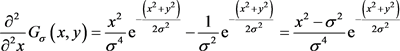(15)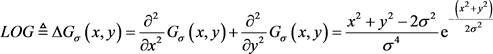(16)(17)

2.4. 二项式系数滤波(18)(19)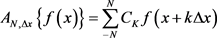(20)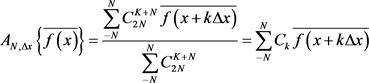(21)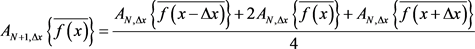(22)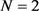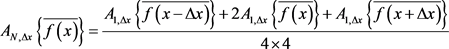3. 实验验证

3.1. 实验对象Table 1. Experimental algorithm comparisonTable 2. Experimental smoothing data

3.2. 实验过程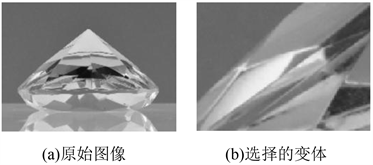Figure 1. Original image and input variantFigure 2. Random noise combination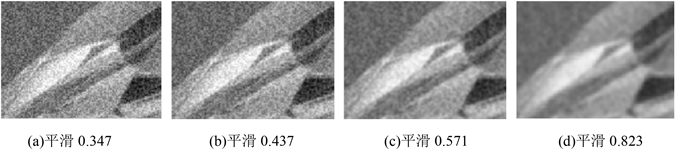Figure 3. Results in IDR with superimposed white noise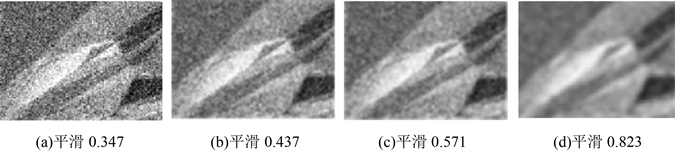Figure 4. Results in IDR with superimposed Gauss noise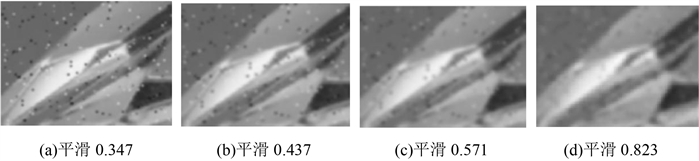Figure 5. Results in IDR with superimposed salt and pepper noiseFigure 6. Results in Gauss filter with superimposed white noiseFigure 7. Results in Gauss filter with superimposed Gauss noise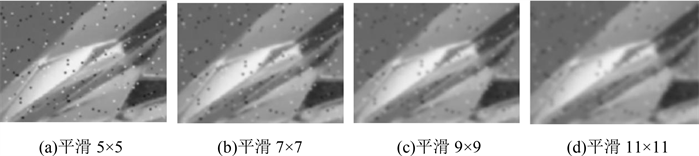Figure 8. Results in Gauss filter with superimposed salt and pepper noise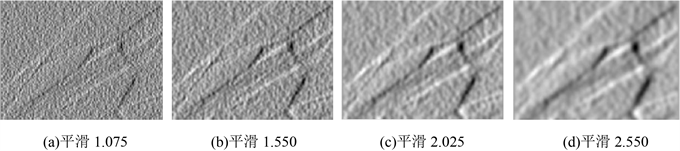Figure 9. Results in LOG with superimposed white noise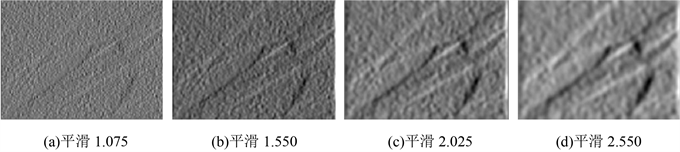Figure 10. Results in LOG with superimposed Gauss noise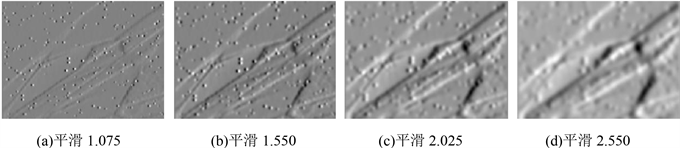Figure 11. Results in LOG with superimposed salt and pepper noise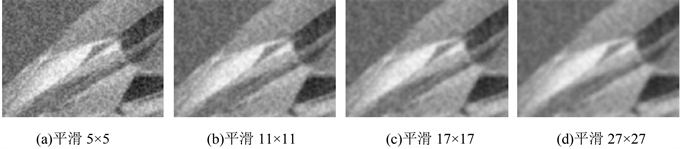Figure 12. Results in BC with superimposed white noise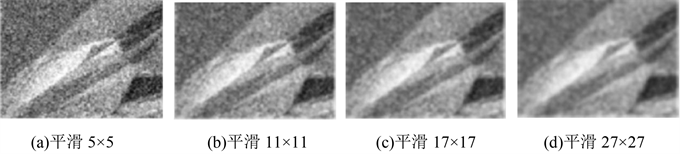Figure 13. Results in BC with superimposed gauss noise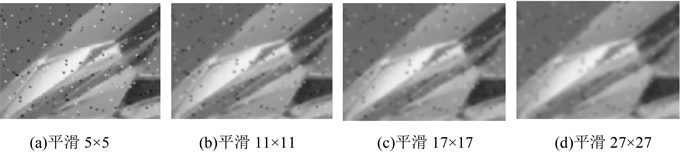Figure 14. Results in BC with superimposed salt and pepper noise

3.3. 结果分析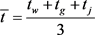(23)Table 3. Calculation time of experimental algorithm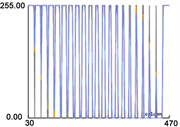(a) 第一组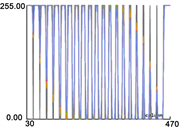(b) 第二组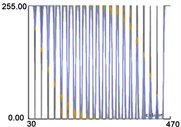(c) 第三组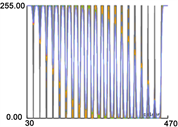(d) 第四组

Figure 15. Gray section of experimental output

4. 结束语

  王耀贵. 图像高斯平滑滤波分析[J]. 计算机与信息技术, 2008(8): 79-81+90.  景晓军, 李剑峰, 熊玉庆. 静止图像的一种自适应平滑滤波算法[J]. 通信学报, 2002, 23(10): 6-14  冯鹏, 魏彪, 米德伶, 潘英俊, 于渝. 基于时域递归滤波的动态数字图像降噪[J]. 重庆大学学报(自然科学版), 2005, 28(2): 23-25+31.  姒绍辉, 胡伏原, 顾亚军, 鲜学丰. 一种基于不规则区域的高斯滤波去噪算法[J]. 计算机科学, 2014, 41(11): 313-316.  Marr, D. and Hildreth, E. (1980) Theory of Edge Detection. Proceedings of the Royal Society of London (Series B), Biological Sciences, 207, 187-217. https://doi.org/10.1098/rspb.1980.0020  严国萍, 何俊峰. 高斯-拉普拉斯边缘检测算子的扩展研究[J]. 华中科技大学学报(自然科学版), 2006, 34(10): 21-23.  彭军还. 数字滤波器的两个理论公式及其应用[J]. 桂林冶金地质学院学报, 1993(4): 405-412.  肖秀春, 彭群生, 卢晓敏, 王章野, 张雨浓, 姜孝华. 基于次序统计量像素灰度相似度的图像双边滤波[J]. 计算机辅助设计与图形学学报, 2011, 23(7): 1232-1237.  王静, 潘梦清. 二项式系数滤波的谱特征[J]. 桂林工学院学报, 2000, 20(4): 379-382.  刘德春, 谭信. 非线性滤波算法性能对比[J]. 电子设计工程, 2011, 19(13): 49-51.